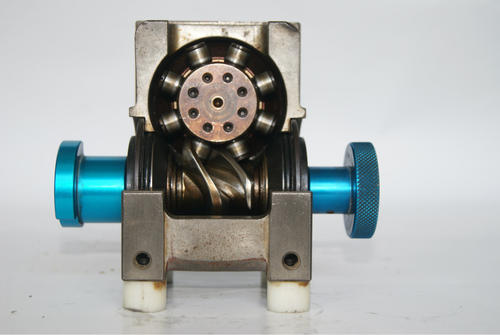分割器

# 凸轮分割器和电机选型计算

下面一起来看看凸轮分割器和电机选型计算：

(1)间歇分割定位等分:S=2 S:分割数

(2)每等分驱动时间：1秒;定位时间：30秒

注：动程时驱动速度比较慢些好，此处按照经验选择动程角(驱动角)270度的分割器德士的6DF，电机选用200W，变速比1:30，再行验算。

(3)输入轴之回转数:N=50rpm，减速箱变比为1：30.(约：N=50Rpm，慢一点并没有问题，电机转速为1500rpm)

(4)凸轮曲线:变形正弦曲线(采用标准普通的就行)

(5)回转盘的尺寸:500×20T ，铝2A12。

(6)夹具的重量:3kg/组 (重量比较复杂，但肯定不到3kg)。

(7)工件的重量:5kg/组 (工件重量比较复杂，但肯定不到5kg)

(8)转盘依靠其底部之滑动面支持本身重量负荷有效半径：R=250mm.

(9)夹具固定于节圆直径330mm处。

计算:

间歇分割定位等分:S=2

输入轴的回转数:N=50rpm

凸输曲线是变形正弦曲线,因此 Vm =1.76 ，Am=5.33 ，Om=0.991，负载扭矩:Tt

(a) 转盘重量:w1;夹具重量：w2;工件重量：w3;则：

w1=10(kg)

w2==6(kg)

w3=10(kg)

(b) 回转盘惯性矩：I1;夹具惯性矩：I2;工件惯性矩：I3;则：

I1=W1R2/(2G)=10x0.252/(2x9.8)=0.032(kg x m x s2)

I2=W2Re2/G=6x0.1652/9.8=0.0167(kg x m x s2)

I3= W3Re2/G=10x0.1652/9.8=0.028(kg x m x s2)

(c)总惯性矩:I

I=I1+I2+I3 I=0.0767(kg x m x s2)

(d)输出轴最大角加速度:

(1) 惯性扭矩:Ti

Ti=I×α=0.0767×21.43=1.644(kg . m)

(2) 摩擦扭矩:Tf

Tf=μ×w×R=0.15*30*0.25=1.125(kg . m)

注意：此处有问题：按照实际的机械设计，分割器转盘只是一边有支撑，另一边是没有支撑的，其摩擦力矩远远没有计算的这么大，或者缩小一倍更为合理。以下先按上面的数值的0.5倍进行计算，校验原来的经验的选型有没有余量。

修正后的Tf=0.5625(kg . m)

(3)作功扭矩:Tw

在间歇分割时没有作功，因此Tw=0

以上总负载扭矩:Tt=Ti+Tf+Tw=1.644+0.5625+0=2.2Kg.m

实际负载扭矩:Te安全负载的因数fe=1.8

Te=Tt×fe=2.2×1.8=3.97(kg . m)

输入轴扭矩:Tc注：输入轴起动负载扭矩视为0. 因此Tca=0

Tc=360/(θh*s) ×Qm×Te=360/(270×2 )×0.99×3.97=1.72(kg. m)

计算所需的马力:p=Tc×n / 716×f(HP) 或 P=Tc×n / 975×f (kw)

假设效率f=60% 那么 P=1.72×50 / 975×0.6 =0.147(Kw) =147W

式中：

Vm: 最大非向性速度

Am: 最大非向性之加速度

Qm: 凸轮轴最大扭力系数

α: 输出轴最大角加速度

θh :驱动角

μ: 磨擦系数=0.15

选择适用的间歇分割器 ：

根据以上所计算的资料以及输入轴的转数50rpm来选择,参考说明书上所记载,凡是输出轴扭矩高于以上所计算的Te值者均可选用。因为Te=3.97 (kg.m),选用法兰型的分割器，按照样本，6DF不够用的(其输出轴最大扭矩为1.41Kg.m),必须采用7DF的分割器(其输出轴最大扭矩为4.3Kg.m)。

分割数：2

动程角：270度;

右旋;

优选正弦曲线，(标准型)。

法兰式，有辅助输出轴，安装定位凸轮用。

安装方式：所以采用(顶面)法兰侧悬挂安装。电机也是悬挂安装。

电机功率：200W，减速器1：30，选用东方电机的带减速箱型号：BHI62EMT-30RH。